# Introduction to Neural Networks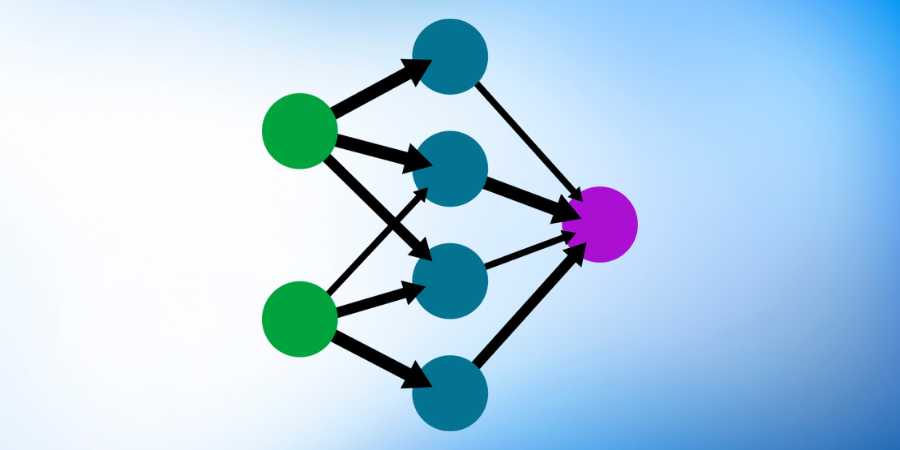S.No Lesson Title
1
##### Introduction
1.1 Artificial Neural Networks
2
2.1 Neuron
2.2 Soma
2.3 Dendrites
2.4 Axon
2.5 Synapse
3
##### Architecture of ANN
3.1 Perceptron
3.2 Activation Function
4
##### Types of Activation Functions
4.1 Threshold Activation Function
4.2 Sigmoid Activation Function - (Binary Step Function)
4.3 Hyperbolic Tangent Function - (Logistic Function)
4.4 Rectified Linear Units - (ReLu)
4.5 Leaky ReLu
5
##### Working of Neural Networks
5.1 Learning of a Neural Network
6

### Introduction

Deep Learning is one of the most powerful branches of Machine learning. Deep learning is a crucial part of the technology involved in creating self-driving cars, which enables them to recognize obstacles or traffic lights, etc. In deep learning, we train a computer model to perform tasks by training on text, image, or audio data. In many cases, deep learning models are able to perform tasks with more accuracy than humans. In deep learning, the models are created using neural networks which often contain many layers.

#### Artificial Neural Networks

An Artificial Neural Network which is abbreviated as ANN is a structure that is inspired by the way our nervous system processes information. An ANN contains a lot of interconnected elements( neurons) which work together to solve a particular problem, just like our brain.

### Human Brain

#### Neuron

In order to understand ANN let us first understand how neurons work in our brain. The biological neurons are the basic unit of our brain and the nervous system. They are the cells, which are responsible for receiving sensory input from the world using the dendrites. The neurons then process these inputs and then provide an output through the axons.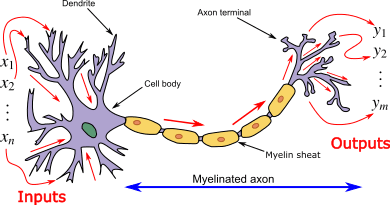#### Soma

It is the cell body of the neuron.

#### Dendrites

Every neuron has very thin, tubular structures around it, which branch out around the cell body. The dendrites are responsible for accepting the incoming signals.

#### Axon

It is a long, thin, tubular structure that works like a transmission line.

#### Synapse

The neurons are connected to each other in a complicated arrangement. At the end of the axon are structures known as synapses, which are responsible for connecting two neurons. The dendrites receive input signals via the synapses of other neurons.

Dendrites receive input through the synapses of other neurons. The soma processes these incoming signals over time and converts that processed value into an output, which is sent out to other neurons through the axon and the synapses.

### Architecture of ANN

#### Perceptron

In the below diagram we can see the general model of an ANN. A neural network with a single layer is called a Perceptron. If it has multiple layers then it is called a Multi-Layer Perceptron.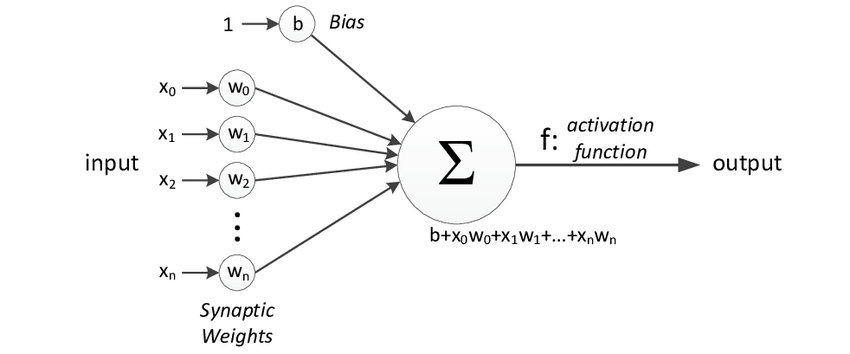In the above figure, we can see that, for a single observation, x0, x1, x2, x3...x(n) represents various inputs to the network. Every single input is multiplied by its corresponding weight which is represented by w0, w1, w2 . . . w(n). Weights show us the strength of a particular node. Now, b is the bias value. A bias value is a value that allows us to shift our activation function up or down.

In the most trivial scenario, all these products are summed together and then fed to an activation function, which generates a result.

Mathematically, x1.w1+x2.w2+.x3.w3..... xn.wn = ∑ xi.wi

Now activation function is applied (∑ xi.wi)

#### Activation Function

The activation function is an integral part of an ANN. The main goal of the activation function is to convert the input signal of a node to an output signal, which is then used as input for the next layer. An activation function helps us to decide whether a neuron should be activated or not by calculating the weighted sum and adding it to the bias. The purpose of this is to inculcate non-linearity in the output of a neuron.

If we don't use an activation function, then the output signal would just be a linear function. The problem with that is, a linear function is limited by its complexity and it has less power. So, without an activation function, our model cannot learn complicated data such as images, audio, etc. Non-Linear functions are the functions that have a degree greater than one and also have a curvature. Now, a neural network can learn almost anything and any complicated function which connects an input to output.

Now let us see some of the activation functions that we have available.

### Types of Activation Functions

#### 1. Threshold Activation Function

In a threshold activation function, if the input value is above or below a particular threshold, then the neuron is activated and it sends the same signal to the next layer.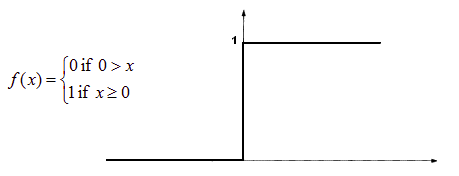#### 2. Sigmoid Activation Function - (Logistic function)

A sigmoid function gives values between 0 and 1. Hence, it is used in models wherein we need to predict the probability as an output.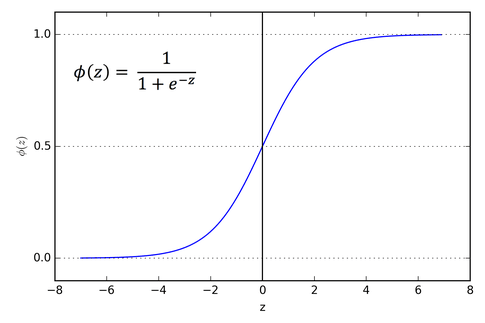#### 3. Hyperbolic Tangential Function - (tanh)

The tanh function is similar to the sigmoid function but it is better in performance. The range of this function is between (-1, 1).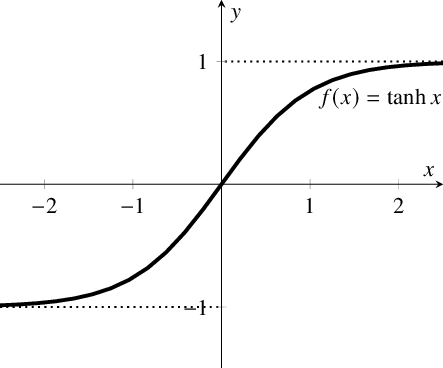#### 4. Rectified Linear Units - (ReLu)>

ReLu is one of the most widely used activation functions while training a CNN or ANN. The value ReLu ranges from zero to infinity.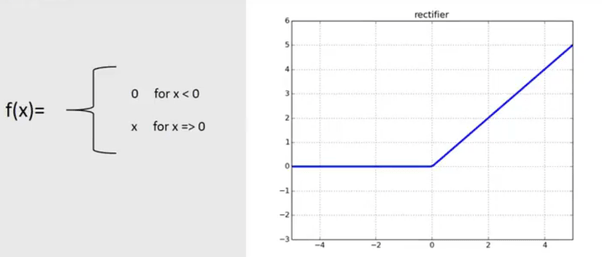#### 5. Leaky ReLu

Leaky ReLu ranges from -∞ to +∞.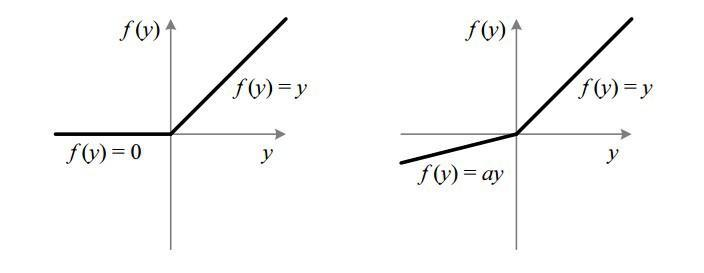Based on our needs, we can decide what activation function we want to use. Now that we know about activation functions, let us now understand how a neural network actually works.

### Working of Neural Networks

Let us understand the working of a neural network by taking the example of the price of a property.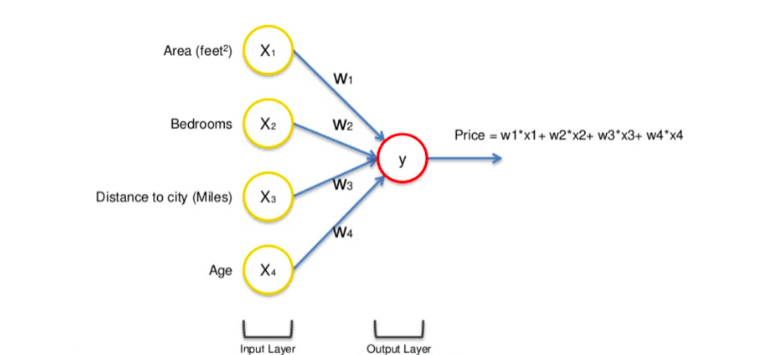Here all the input values X1, X2, X3, and X4 go through the weighted neurons of the input layer. After this, all 4 values go to the output layer. They are analyzed and an activation function is applied to them, after which the result is produced.

We can further increase the power of the neural network by adding hidden layers which are located between the input and output layers.A neural network with a hidden layer(only showing non-0 values)

Now here we can see that all 4 variables are connected to the neurons through a synapse. But, not all synapses will have a weight.

If weight is 0 then it will be discarded, and a non 0 value of weight will indicate the importance. Let us understand this by taking the variables Bedrooms and Age to be non-zero for the first neuron. This means that they are weighted and they matter to the first neuron. The remaining variables which are Area and Distance to the city are not weighted and hence they are not taken into consideration by the first neuron.

This is one of the main reasons that neural networks are so powerful. There are many such neurons in a network and each of them is doing calculations like this. Once the calculation is done, the neuron applies the activation function and does its final calculations. This way the neurons work and look for specific things while training.

#### Learning of a Neural Network

To understand how a neural network learns, let us take a closer look at how human beings learn. We perform a task, and it is either appreciated or corrected by a trainer/teacher so that we can understand how to get better at that task. In a similar way, neural networks need a trainer which can describe what should be produced as a response to the input. On the basis of the actual value and predicted value, an error is calculated and it is sent back through the system. This error value is also called the cost function.

For every layer in the network, the cost function is calculated, and based on that value, the weights are adjusted for the next input. Our goal in this is to minimize the cost function because the lower the cost function would be, the greater will be the similarity between the actual and the predicted values. So as the network learns, the error keeps on reducing.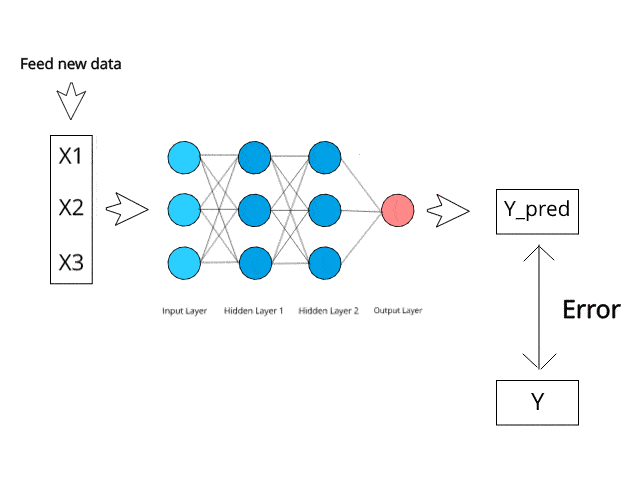We feed the resulting data back through the entire neural network. The weighted synapses connecting input variables to the neuron are the only thing we have control over.

As long as we have a difference between the actual and the predicted values, we need to keep adjusting the weight. After we update the weights, we run the neural network again on our data. This will give us a new cost function. We will send this cost function back again. This process of sending the cost function back is called back-propagation. We need to back-propagate the loss until our loss is as small as possible.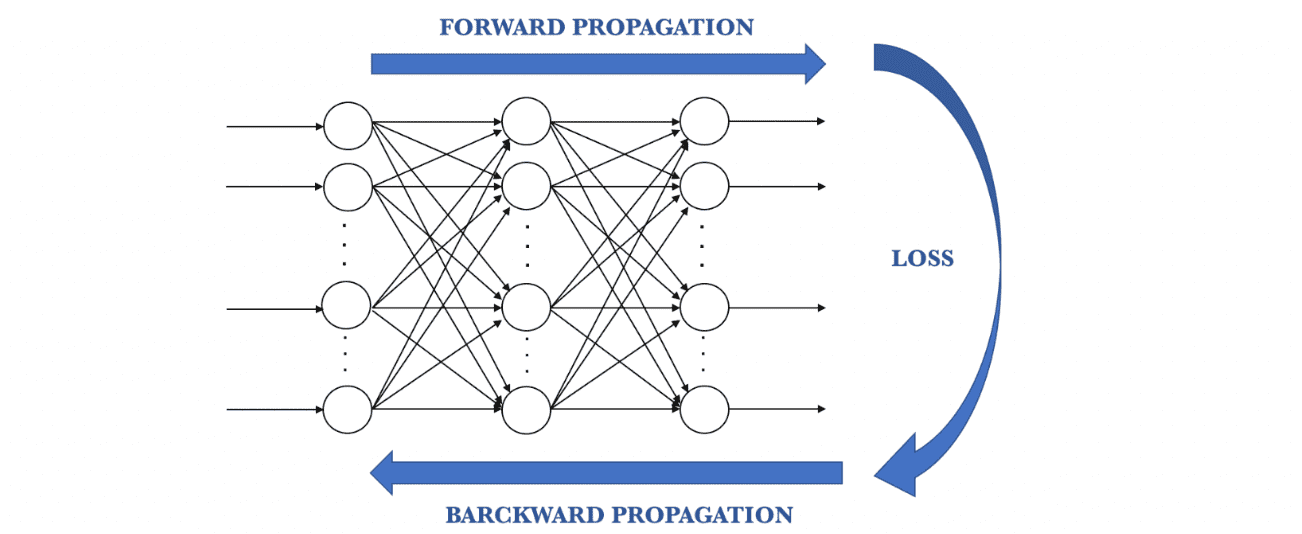### Conclusion

In this article, we have briefly explained the idea behind the working of neural networks. Further, we have also explored different activation functions that are used in building the models. We believe this article helps to get the coincide overview of the mechanism of neural networks.

Send Message
Top